# ISEE Upper Level Math : Operations

## Example Questions

### Example Question #6 : How To Add Variables

Simplify the following: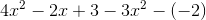Possible Answers: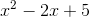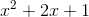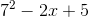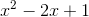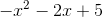Correct answer:Explanation:

To simplify this expression, you need to combine like terms.

There are two terms with, one term with, and two terms without a variable.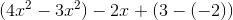This gives you the final answer:Remember that when you subtract by a negative number, you are actually adding the inverse - thereby adding a positive number.

### Example Question #7 : How To Add Variables

Simplify the following: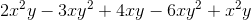Possible Answers: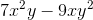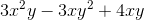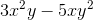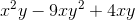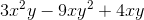Correct answer:Explanation:

In this problem, you need to combine like terms. Be very careful when combining like terms, since a few terms differ only by a few exponents. You have two terms with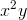, two terms with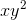, and one term with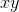.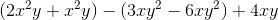This leads to the final answer:### Example Question #2 : How To Add Variables

Multiply: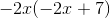Possible Answers: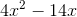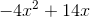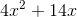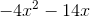Correct answer:Explanation: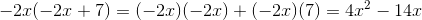### Example Question #9 : How To Add Variables

Simplify: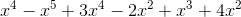Possible Answers: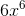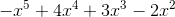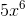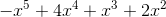Correct answer:Explanation:

Combine like terms: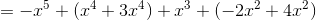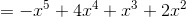### Example Question #10 : How To Add Variables

If two supplementary angles are equal toand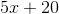, then what is the value of?

Possible Answers:Correct answer:Explanation:

The sum of two supplementary angles is always 180 degrees; therefore: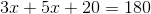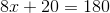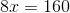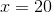### Example Question #51 : Operations

On Gina's swim team, 40 percent of the swimmers also play one other sport (cross country, soccer, or baseball) competitively. There are 20 people on her swim team. Of the swimmers who play one other sport, 2 of them participate in cross country, and twice as many people play soccer as those who play baseball.  How many play soccer?

Possible Answers:Correct answer:Explanation:

On Gina's swim team, 40 percent of the swimmers also play one other sport competitively. Given that there are 20 people on her swim team, 8 people play one other sport because 40 percent of 20 is 8.

If 2 swimmers run cross country that leaves 6 swimmers who play a different sport.

Given that twice as many play soccer as those who play baseball, it follows that 4 play soccer and 2 play baseball.

Thus, 4 is the correct answer.

### Example Question #942 : Isee Upper Level (Grades 9 12) Mathematics Achievement

Simplify the following expression: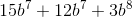Possible Answers: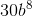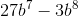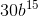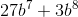Correct answer:Explanation:

Simplify the following expression:We can solve this question in one step.

We need to add some variables, but we need to recall that we can only add variables with the same exponent. Thus, we can only add the b^7's.

With this in mind, simply add the coefficients and keep the rest the same.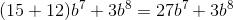So our answer is### Example Question #943 : Isee Upper Level (Grades 9 12) Mathematics Achievement

Simplify the following expression.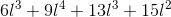Possible Answers: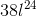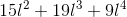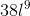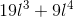Correct answer:Explanation:

Simplify the following expression.When adding variables, we can only add those with the same exponent. The others must remain unchanged.

That means that in this problem, we can only add the blue terms.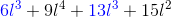Because these terms are the only ones with the same exponents.

So, to add them, keep the exponents the same and add the coefficients (the numbers out in front).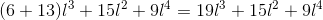Now, just rewrite it in standard form and you have our answer### Example Question #944 : Isee Upper Level (Grades 9 12) Mathematics Achievement

Combine the following: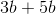Possible Answers: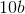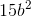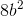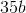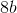Correct answer:Explanation:

When adding variables, we will look at the variables like objects.

So, in the problemwe can think of the variable b as books.  So,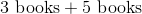We can read it like this:  We borrowed 3 books from the library yesterday.  We go back to the library and borrow 5 more books.  How many books have we borrowed altogether?  We have borrowed 8 books.  So,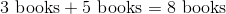We add variables the same way.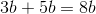### Example Question #945 : Isee Upper Level (Grades 9 12) Mathematics Achievement

Simplify the following: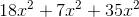Possible Answers: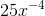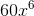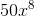Correct answer:Explanation:

Simplify the following:To combine the given expression, we need to realize that we can only combine terms with the same exponent.

In this case, all our terms have the same exponents, so we can add them up just like we would any integer.

To add up our terms, simply add up the coefficients and keep the exponent the same. (With this in mind we could eliminate any answer choices which do not have an exponent of two)

So, do the following: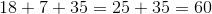\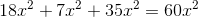So, we have our answer of 60 x squared

### All ISEE Upper Level Math Resources